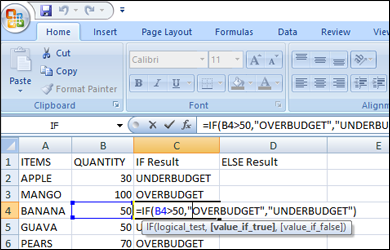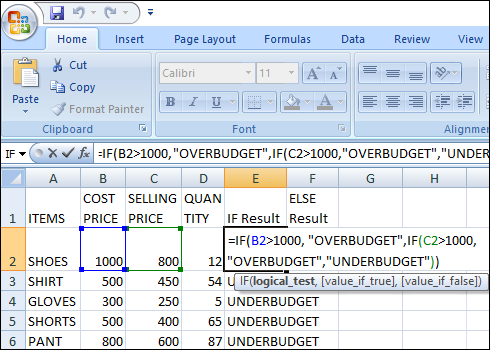# IMPLEMENTATION OF MULTIPLE IF STATEMENTS

Updated On:

Excel allows user to implement if-statements in multiple formats which are mentioned below.

1. IF-FUNTION:• If I am having a data as excel sheet which contains two parameters i.e. items and its quantity.
• Select the desired cell if you want to apply if-statement on the particular cell of available data.
• Syntax for if-statement is =IF (Condition, “return-true-value”, “return-false-value”).
• Implement this mentioned formula in IF Result column =IF (B2>50, “OVERBUDGET”, “UNDERBUDGET”). Where B is the column for quantity of items. If the above condition is true then, the final result will be OVERBUDGET otherwise it will show UNDERBUDGET.
• Press enter to view the final result.
• You can also implement the same formula on all the cells by dragging it to other cells.

3. IF-STATEMENT WITH OR OPERATOR:

• If I am having a data as excel sheet which contains two parameters i.e. items and its quantity.
• Select the desired cell if you want to apply if-statement on the particular cell of available data.
• Syntax for if-statement is IF (Condition, “return-true-value”, “return-false-value”).
• Implement this mentioned formula in IF Result column =IF (B2>50, “OVERBUDGET”, “UNDERBUDGET”). Where B is the column for quantity of items. If the above condition is true then, the final result will be OVERBUDGET otherwise it will show UNDERBUDGET.
• Press enter to view the final result.
• You can also implement the same formula on all the cells by dragging it to other cells.

2. IF-STATEMENT WITH AND OPERATOR:

• Suppose that you are having a data in excel with three parameters i.e. Items, Price and Quantity and you want to operate it by If-Statement with AND Operator.
• Then, Select the desired cell to operate if function with AND.
• Use this Syntax for AND =IF (AND (condition1, condition2, condition3………), return-value-if-true, return-value-if-false).
• Implement this formula on selected cell =IF (AND (B2>50, C2>10, “DELIVER”, “UNABLE TO DELIVER”). Where B and C is the price and quantity of items respectively. This formula will return DELIVER if all the conditions are true otherwise it will give UNABLE TO DELIVER as a result.
• Click enter button to view the result.
• You are also allowed to add this formula to multiple cells.
4. IF-STATEMENT WITH ARAAY:
• This one is totally based on arrays where we are able to fill rest of the cells only by knowing the first cell of the record. Arrays are having much syntax for multiple operations to perform on arrays. You are allowed to have sum, average, product and many more operations to implement on arrays using if-statement. Array is the concept which allows user to perform operations on multiple values at the same time.
• You can use array formula inside if-statement to find out the overall result of the record.
• Create the data record in excel first then, apply array formula on a particular range as described in the steps mentioned below.
• Syntax for sum in if-statement with arrays is: {=SUM (B2:B6*C2:C6)}.Where B and C are the quantity and price of items. This formula will calculate the sum of column B and also sum for C column then, product both the values to throw the final result. Where B and C is the price and quantity of items respectively. This formula will return DELIVER if all the conditions are true otherwise it will give UNABLE TO DELIVER as a result.
• If-statements also help us to find the maximum value by using array formula i.e. {=MAX (B2:B6-C2:C6)}. Where B and C are the quantity and price of items. This will give you the maximum increase in sales.
• Syntax of adding data in a row by using array is {={A,B,C,D}}.
5. IF-STATEMENT WITH MULTIPLE AND & OR:
• Create the data in excel according to your needs. I am having an excel sheet with items their Cost and selling price and quantity also..
• Select the desired cell from the same data which you want to operater.
• Start operating it with If function having multiple AND & OR.
• Syntax for multiple AND & OR operator is there i.e. =IF (OR (AND (condition1, condition2)), (AND (condition3, condition4)), return-value-if-true, return-value-if-false).
• Use this mentioned syntax for this operation i.e. =IF (OR (AND (B2>=50, D2>=10)), (AND (C2>=500, D2>=20)), “POSSIBLE DELIVERY”, “SORRY UNABLE TO DELIVER”).
• Final step is to view the result by pressing Enter button.
6. NESTED IF-STATEMENT:• Suppose you are having a data containing fruits as items and their cost and selling price and quantity as well.
• If you want to operate a cell by nested if then, you must sue this syntax =IF (condition1, “return-true-value”, IF (condition2, “return-true-value”, “return-false-value”)).
• Choose the cell from the available data and use the syntax mentioned in next step.
• =IF (B5>1000, “OVERBUDGET”, IF (C5>1000, “OVERBUDGET”, “UNDERBUDGET”)).
• Last step is to click Enter to view the final result.
7. IF-STATEMENT WITH NOT OPERATOR:
• 1. the data in excel to operate if-statement with NOT operator.
• 2. Select the desired cells to operate NOT.
• 3. Use the syntax mentioned below to operate if function with NOT operator.
• 4. Use this syntax to apply Not operator =IF (NOT (CONDITION, “return-true-value”, “return-false-value”)).
• 5. Example to implement this operator in excel is mentioned =IF (NOT (B2=50, “TRUE”, “FALSE”)). Where B is the column for quantity of items. If above condition is TRUE then, NOT will return False and it will return TRUE if it is wrong.
• 6. Click Enter button to view the result.

### HOW IF-STATEMENT IS BENEFICIAL:

1. IF-Statement helps to evaluate the greater, smaller, less than, greater than, equal to by using the set of various conditions.
2. If function is beneficial to have logical comparison between two or more values.
3. If-statement empowers user with nested if to determine the data in a range. Basically, it is the test for combination of various conditions to make a final record.